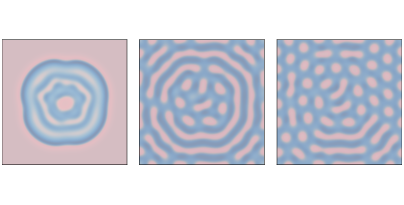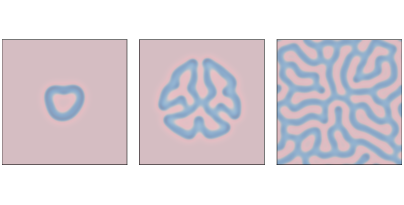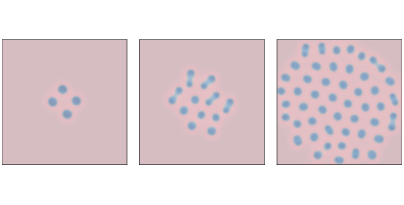# 9.3. Reaction-Diffusion¶

Now let’s add a second chemical. We will define a new object, `ReactionDiffusion`, that contains two arrays, one for each chemical:

``` class ReactionDiffusion(Cell2D):

def __init__(self, n, m, params, noise=0.1):
self.params = params
self.array = np.ones((n, m), dtype=float)
self.array2 = noise * np.random.random((n, m))
```

`n` and `m` are the number of rows and columns in the array. `params` is a tuple of parameters, which is explained below.

`array` represents the concentration of the first chemical, `A`; the NumPy function `ones` initializes it to 1 everywhere. The data type `float` indicates that the elements of `A` are floating-point values.

`array2` represents the concentration of `B`, which is initialized with random values between 0 and `noise`, which is 0.1 by default. Then `add_island` adds an island of higher concentration in the middle:

```def add_island(a, height=0.1):
n, m = a.shape
radius = min(n, m) // 20
i = n//2
j = m//2
```

The radius of the island is one twentieth of `n` or `m`, whichever is smaller. The height of the island is `height`, with the default value 0.1.

Here is the `step` function that updates the arrays:

```def step(self):
A = self.array
B = self.array2
ra, rb, f, k = self.params

cA = correlate2d(A, self.kernel, **self.options)
cB = correlate2d(B, self.kernel, **self.options)

reaction = A * B**2
self.array += ra * cA - reaction + f * (1-A)
self.array2 += rb * cB + reaction - (f+k) * B
```

The parameters are:

ra: The diffusion rate of `A` (analogous to `r` in the previous section).

rb: The diffusion rate of `B`. In most versions of this model, `rb` is about half of ra.

f: The “feed” rate, which controls how quickly `A` is added to the system

k: The “kill” rate, which controls how quickly `B` is removed from the system.

Now let’s look more closely at the update statements:

```reaction = A * B**2
self.array += ra * cA - reaction + f * (1-A)
self.array2 += rb * cB + reaction - (f+k) * B
```

The arrays `cA` and `cB` are the result of applying a diffusion kernel to `A` and `B`. Multiplying by `ra` and `rb` yields the rate of diffusion into or out of each cell.

The term `A * B**2` represents the rate that `A` and `B` react with each other. Assuming that the reaction consumes `A` and produces `B`, we subtract this term in the first equation and add it in the second.

The term `f * (1-A)` determines the rate that `A` is added to the system. Where `A` is near 0, the maximum feed rate is `f`. Where `A` approaches 1, the feed rate drops off to 0.

Finally, the term `(f+k) * B` determines the rate that `B` is removed from the system. As `B` approaches 0, this rate goes to 0.

As long as the rate parameters are not too high, the values of `A` and `B` usually stay between 0 and 1.Figure 9.2: Reaction-diffusion model with parameters f=0.035 and k=0.057 after 1000, 2000, and 4000 steps.

With different parameters, this model can produce patterns similar to the stripes and spots on a variety of animals. In some cases, the similarity is striking, especially when the feed and kill parameters vary in space.

For all simulations in this section, `ra=0.5` and `rb=0.25`.

Figure 9.2 shows results with `f=0.035` and `k=0.057`, with the concentration of `B` shown in darker colors. With these parameters, the system evolves toward a stable configuration with light spots of `A` on a dark background of `B`.Figure 9.3: Reaction-diffusion model with parameters f=0.055 and k=0.062 after 1000, 2000, and 4000 steps.

Figure 9.3 shows results with `f=0.055` and `k=0.062`, which yields a coral-like pattern of `B` on a background of `A`.Figure 9.4: A reaction-diffusion model with parameters f=0.039 and k=0.065 after 1000, 2000, and 4000 steps.

Figure 9.4 shows results with `f=0.039` and `k=0.065`. These parameters produce spots of `B` that grow and divide in a process that resembles mitosis, ending with a stable pattern of equally-spaced spots.

Since 1952, observations and experiments have provided some support for Turing’s conjecture. At this point it seems likely, but not yet proven, that many animal patterns are actually formed by reaction-diffusion processes of some kind.